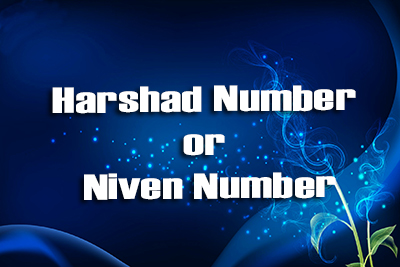FREE ASSISTANCE FOR THE INQUISITIVE PEOPLE
Tutorial Topics
Pronic Number - java Disarium Number
Harshad Number or Niven Number - Java
12457    Arnab De    10/12/2018Write a Program in Java to input a number and check whether it is a Harshad Number or Niven Number or not..

Harshad Number : In recreational mathematics, a Harshad number (or Niven number), is an integer (in base 10) that is divisible by the sum of its digits.

The number 18 is a Harshad number in base 10, because the sum of the digits 1 and 8 is 9 (1 + 8 = 9), and 18 is divisible by 9 (since 18 % 9 = 0)

The number 1729 is a Harshad number in base 10, because the sum of the digits 1 ,7, 2 and 9 is 19 (1 + 7 + 2 + 9 = 19), and 1729 is divisible by 19 (1729 = 19 * 91)

The number 19 is not a Harshad number in base 10, because the sum of the digits 1 and 9 is 10 (1 + 9 = 10), and 19 is not divisible by 10 (since 19 % 10 = 9)

Logic : Divide the actual number by the sum of the digits of actual number. if it is divisible then print a positive message otherwise print a negative message

```import java.util.*;
{
public static void main(String []args)
{
Scanner sc=new Scanner(System.in);
System.out.print("Enter a Number : ");
int no=sc.nextInt();
int s=0,temp=no;
while(temp>0)
{
s += temp%10;
temp /= 10;
}
if(no%s==0)
{
System.out.println("This is a Harshad no or Niven No.");
}
else
{
System.out.println("This is not a Harshad no or Niven No.");
}
}
}
```
Pronic Number - java Disarium Number
Author DetailsArnab De
I have over 16 years of experience working as an IT professional, ranging from teaching at my own institute to being a computer faculty at different leading institute across Kolkata. I also work as a web developer and designer, having worked for renowned companies and brand. Through tutorialathome, I wish to share my years of knowledge with the readers.
Enter New Comment
Comment History
No Comment Found Yet.What makes one man great and another weak and low is shraddha (concentration).
Swami Vivekananda
952
44.84
Today So Far
Total View (Lakh)